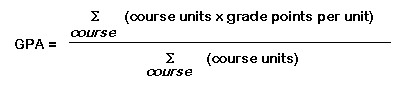# GPA conversion

### Grade Point Average Computation

We require that each applicant compute his/her own grade point average (GPA) from the formula:Please use the worksheet provided below and bear in mind the following specifications:

1. 1. The courses to be included in the computation of your GPA are all courses for which a grade has been awarded (both overall and in your last two years). If your transcript contains your GPA, that is sufficient. However, it should be converted to the four-point system.
2. 2.  The four-point system means that a 4 grade point per unit corresponds to a grade of “A” (outstanding), 3 points to be a “B” (good), 2 points to a “C” (satisfactory), 1 point to a “D” (barely passing) and 0 points for an “F” grade (failing). If your university assigns plus and minus grades, these then are to be used in the computation with + = +0.3 and -=-0.3. Thus, if a “B” is 3.0, a “B+” is 3.3 and a “B-” is 2.7. (Please note that the University of California does not recognize the letter grade of “A+”, i.e., “A+” is 4.0!
3. 3.  If your institution uses a grading system based on percentage or class honors, please use the following class rank percentages to convert each class evaluation to a letter grade and then proceed as above to compute your equivalent four-point system GPA.